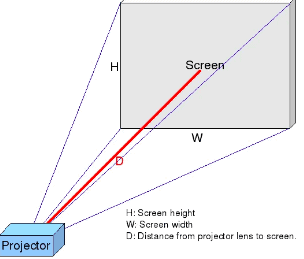# What You Need To Know About Throw Ratio

## Placement dictates size….

### What’s a throw ratio?

Throw ratio is the distance between the projector and the screen in relation to the width of the image being projected.

### How do you determine throw ratio?

By dividing the distance between the projector and the screen, in relation to the width of the image being projected. That calculation equals your throw ratio.

#### Example

If a projector is placed 10 feet away from the screen and the image it projects is 5 feet wide, the throw ratio is 2:1 (10 divided by 5).

### Why does it matter?

The throw ratio determines the size of the image that can be projected from given distances. Therefore, you need to know the @throw ratio!